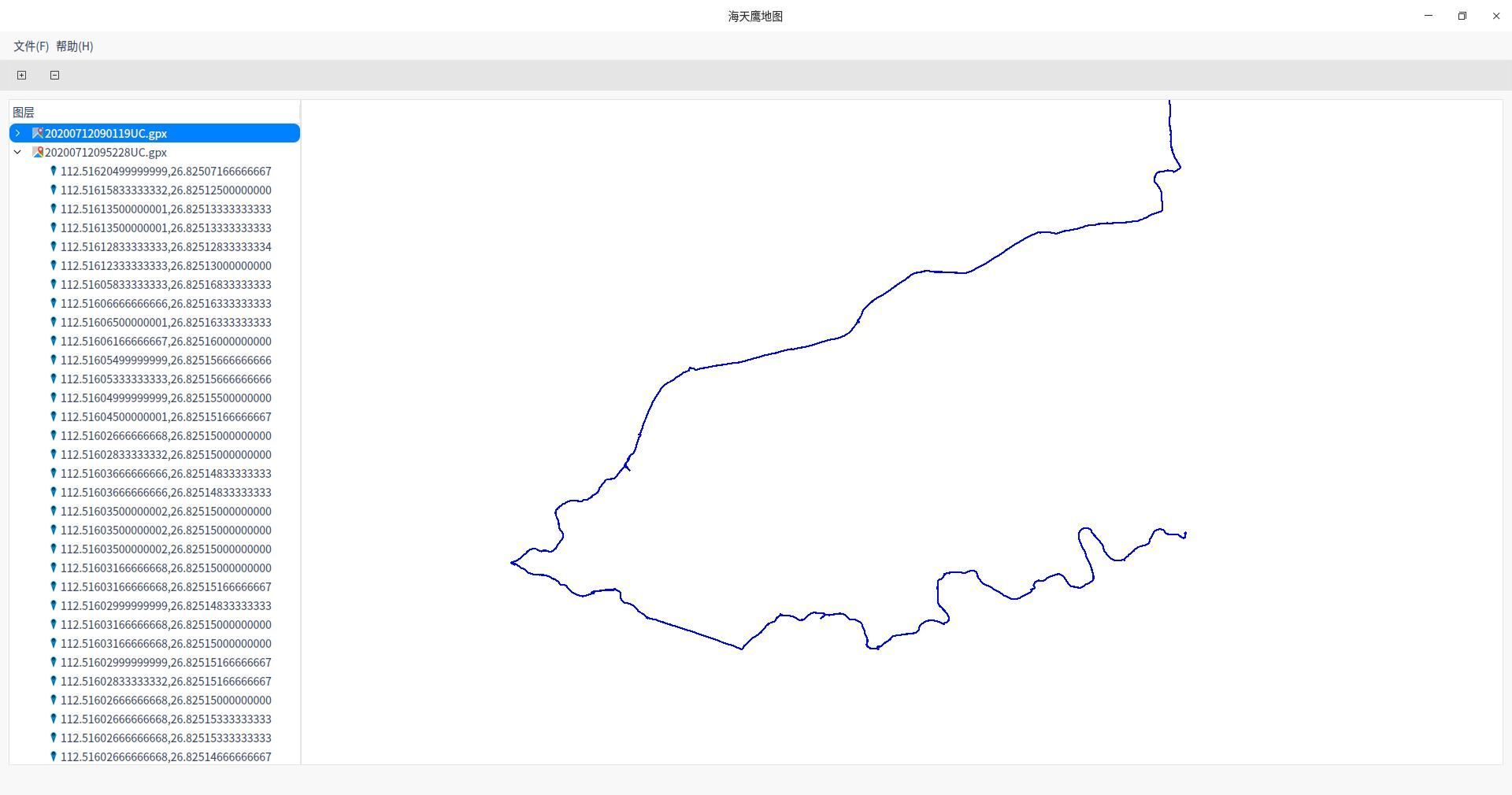# How to change (longitude, latitude) to QGraphicsItem(x,y) ?

• I have already get the point(longitude, latitude) of gpx file, how to draw them on QGrahpicsScene ?``````QFile file(filePath);
return;
QDomDocument doc;
if (!doc.setContent(&file)) {
file.close();
return;
}
file.close();

QTreeWidgetItem *TWI_root = new QTreeWidgetItem(ui->treeWidget);
TWI_root->setText(0, QFileInfo(filePath).fileName());
TWI_root->setIcon(0, QIcon::fromTheme("application-gpx+xml", QIcon(":/icon.png")));

QDomElement root = doc.documentElement();
QDomNodeList DNL = root.elementsByTagName("trkpt");
QPainterPath PP;
for (int i=0; i<DNL.count(); i++) {
QDomNode DN = DNL.at(i);
if (DN.isElement()) {
QDomElement DE = DN.toElement();
qreal lon = DE.attribute("lon").toDouble();
qreal lat = DE.attribute("lat").toDouble();
QTreeWidgetItem *TWI_point = new QTreeWidgetItem(TWI_root);
TWI_point->setText(0, QString("%1").arg(lon, 0, 'f', 14) + "," + QString("%1").arg(lat, 0, 'f', 14));
TWI_point->setIcon(0, QIcon(":/marker.png"));
QGraphicsEllipseItem *GEI = new QGraphicsEllipseItem(lon, lat, 5, 5);
GEI->setFlags(QGraphicsItem::ItemIsSelectable);
if (i == 0)
PP.moveTo(lon, lat);
else
PP.lineTo(lon, lat);
}
}
QPen pen(Qt::blue, 1);
GPI->setFlags(QGraphicsItem::ItemIsSelectable);
``````

• @sonichy

Hi,

do you want to translate your lat / long value to coordinates (x, y values) of your scene?

https://stackoverflow.com/questions/14034455/translating-lat-long-to-actual-screen-x-y-coordinates-on-a-equirectangular-map

• @Pl45m4 The method is not fit.
The key problem is the number is too small, I find another way : Differential Amplification. The shape is correct.

``````QDomElement root = doc.documentElement();
QDomNodeList DNL = root.elementsByTagName("trkpt");
QPainterPath PP;
qreal lonc=0, latc=0;
for (int i=0; i<DNL.count(); i++) {
QDomNode DN = DNL.at(i);
if (DN.isElement()) {
QDomElement DE = DN.toElement();
qreal lon = DE.attribute("lon").toDouble();
qreal lat = DE.attribute("lat").toDouble();
if (i == 0) { // first point as reference point
lonc = lon;
latc = lat;
} else { // Differential then Amplification
qreal x = (lon - lonc) * 10000;
qreal y = - (lat - latc) * 10000; // direction is opposite, add -
PP.lineTo(x, y);
}
}
}
QPen pen(Qt::blue, 1);